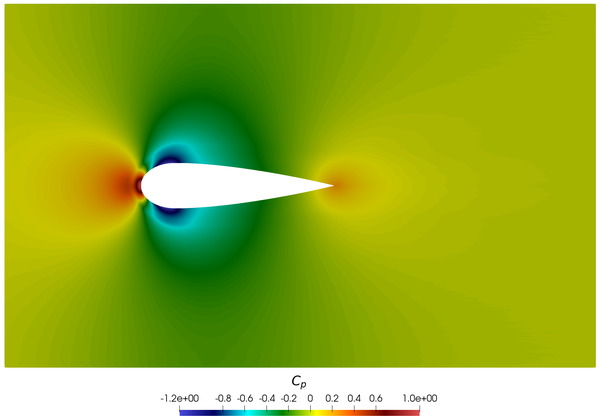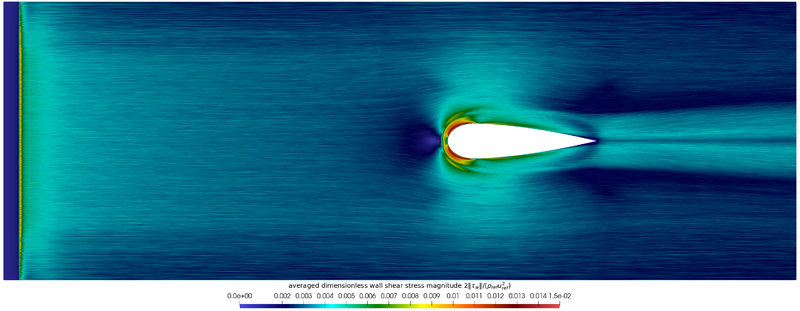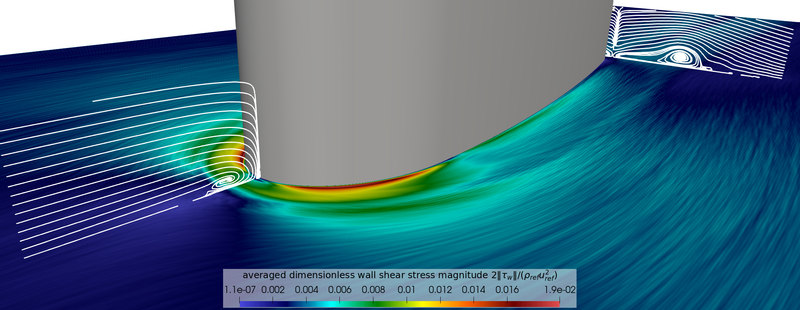# DNS 1-6 Statistical Data

(diff) ← Older revision | Latest revision (diff) | Newer revision → (diff)

# Statistical data

In this section the relevant statistical data for the flow around the wing-body junction computed with MIGALE is given. The reported data is the one mentioned in Table 1 of the list of desirable quantities (PDF).

The data is available as:

• In .vtu (ASCII) format for statistical data.
• In .csv (text) format as vertical profiles at various locations.

This database is a first attempt of DNS for the present wing-body junction flow problem. Although affected by some spatial under-resolution and the need of a larger time window for the collection of the statistics, the information provided is of primary importance for future computational studies. The database will be further studied in the near future and additional results added later.

## Volume data

Volumetric data of the statistics are provided here. For more information regarding the stored quantities and the storage format, please refer to the storage format guidelines.

The available files are:

## Surface data

Surface data of the statistics are provided here. For more information regarding the stored quantities and the storage format, please refer to the storage format guidelines.

The available files are:

The pressure coefficient contour on the bottom surface is reported in Fig. 13. Due to the flow geometry, a favourable pressure gradient is generated downstream of the leading edge, where the flow goes around the wing profile, resulting in a reduction of $C_{p}$(blue area). This reduction attenuates when moving away from the airfoil, but it is still appreciable along the lateral boundaries, i.e., $\max {(C_{p,lateral})}=-0.15$. Accordingly, although small, a blockage effect on the flow due to the lateral boundaries is present. As expected for a flow in the incompressible regime (here $Ma=0.078$), the maximum value of $C_{p}$is close to 1 at the leading edge of the wing.

Fig. 14 shows the time averaged dimensionless wall shear stress distribution on the floor. It is conceivable that the streaky behaviour of the wall shear stress can be ascribed to the need for a larger time window to collect averages.

The favourable pressure gradient on the side of the airfoil nose induces a flow acceleration, which is the main cause of the increase of the wall shear stress in this region. Downstream of the wing, in the wake region, the traces of the horseshoe vortices on the floor can also be observed. The horseshoe vortex can also be seen from the streamlines shown at selected planes in Fig. 15.Figure 13: Wing-body junction. Contour of pressure coefficient on the bottom surface for $-3Figure 14: Wing-body junction. Contour of averaged dimensionless wall shear stress on the bottom surface for $-12.75Figure 15: Wing-body junction. Streamlines at selected planes, i.e., symmetry plane (streamlines generated with $x$- and $y$- components of the averaged velocity field) and at a cross section downstream of the wing (streamlines generated with $y$- and $z$- components of the averaged velocity field) at $x/T=4.46$, superimposed on contours of averaged dimensionless wall shear stress on the bottom surface.

## Profile data

Profile data have been extracted from the symmetry plane $z/T=0$at different streamwise locations ($x/T=-0.45,-0.40,-0.35,-0.30,-0.25,-0.20,-0.15,-0.10,-0.05,-0.01$) and made dimensionless with respect to reference quantities ($u_{ref}$, $\rho _{ref}$). The data stored in each file are:

• vertical location $y/T$• average velocity components $U_{i}/u_{ref}$• Reynolds stress components $R_{ij}/(\rho _{ref}u_{ref}^{2})$• turbulent kinetic energy $k/u_{ref}^{2}$Profiles at selected streamwise locations are reported in Fig. 16.

Table 4: Wing-body junction. Profiles on symmetry plane at different streamwise locations

## Contour data

Contour data of the averaged velocity components $U$and $V$, the three normal stresses $R_{xx}$, $R_{yy}$and $R_{zz}$, the turbulent kinetic energy $k$, and the shear stress $R_{xy}$in the symmetry plane are provided in Fig. 17. As the region depicted is in front of the leading edge, the effect of the horseshoe vortex is visible: while the streamwise component of the velocity becomes negative above the bottom wall, the vertical component shows a large negative region close to the leading edge. This results in a clockwise rotating vortex, the origin of the horseshoe vortex. Within this vortex the Reynolds stresses as well as the turbulent kinetic energy reach maximum values. Moreover, looking more in detail, one can notice the presence of a tiny vortex with anticlockwise rotation clinging to the leading edge of the wing, very near the wing-body junction. Such a small counterrotating vortex was also observed in previous studies of flow around surface-mounted cylinders. The effect of this small vortex can also be seen in the profiles in Fig. 16 at the position closest to the leading edge All contours are normalized with respect to the reference quantities.

Contributed by: Francesco Bassi (UNIBG), Alessandro Colombo (UNIBG), Francesco Carlo Massa (UNIBG), Michael Leschziner (ICL/ERCOFTAC), Jean-Baptiste Chapelier (ONERA) — University of Bergamo (UNIBG), ICL (Imperial College London), ONERA